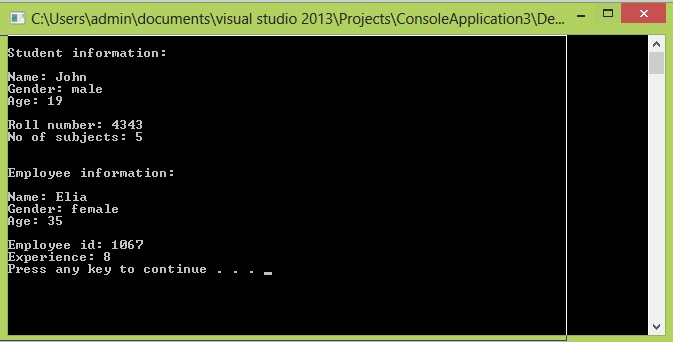# Polymorphism

In this part you will learn: 1. What is polymorphism 2. Why need polymorphism 3. What is virtual function 4. What is pure virtual function 5. Program 6. Output Polymorphism Polymorphism means having many forms. In c++, when we are dealing with classes and their inheritance, there occurs a case when we have more than one derived classes of the base class. Using polymorphism we can call a member function, which will, depending upon the type of object that invokes the function, cause a different function to perform. Polymorphism makes the program simple. Instead of calling different functions for different objects, using polymorphism we can use a base pointer to point to the object of the derived class. When we will call the function, the function defined in that object will be executed automatically. So it will make our program easy to work on. Virtual functions The concept of polymorphism is implemented using virtual functions. In virtual functions, we first declare the function as a virtual function. By this declaration, when the program will reach here and see that it is a virtual function. The program will try looking in the derived class for the overriding function of it. If there is no definition in the derived class, then the virtual function will be called. In general, when a function is declared as virtual, the program tries to reach till the derived class of which the object is made, the last function found is executed. syntax virtual return_type function_name(parameters) { //function definition } Pure Virtual functions By making a function pure virtual, the programmer is bound to define that function in the derived class, otherwise there would be an error in the code. Making a function pure virtual, we are not bound to define that function in the base class instead we just make it equivalent to zero. Syntax virtual return_type function_name(parameters)=0 { //can be empty } Program with polymorphism In the following example, I have coded the working of a school management system using polymorphism. Basic Step:
`#include<iostream>#include<conio.h>using namespace std;`
These two are the most common and basic lines of a c++ program. The first one iostream is used to grant us most of the basic functions of C++ like Input and Output functions. The second one conio.h means console input output, the black screen we see is the console. Using namespace std; provides us an ease of using all the library functions without specifying that this function belongs to standard library. Without including it, we have to write ‘std’ with all the built-in functions. Class
`class person{protected:			//access specifier 				string name;	string gender;	int age; public:	person(string n, string g, int a) :name(n), gender(g), age(a){	}	virtual void print_info() = 0;}; class student :public person{public:			//access specifier 				int roll_no;	int no_of_subjects; 	student(string n, string g, int a, int r, int no) :person(n, g, a), roll_no(r), no_of_subjects(no){	}	void print_info(){		cout << endl << "Student information:" << endl << endl;		cout << "Name: " << name << endl;		cout << "Gender: " << gender << endl;		cout << "Age: " << age << endl << endl;		cout << "Roll number: " << roll_no << endl;		cout << "No of subjects: " << no_of_subjects << endl;	} }; class employee :public person{public:	int employee_id;	int experience; 	employee(string n, string g, int a, int e_id, int e) :person(n, g, a), employee_id(e_id), experience(e){	}	void print_info(){		cout << endl << endl << "Employee information:" << endl << endl;		cout << "Name: " << name << endl;		cout << "Gender: " << gender << endl;		cout << "Age: " << age << endl << endl;		cout << "Employee id: " << employee_id << endl;		cout << "Experience: " << experience << endl;	}};`
In the classes part, there are three classes namely person, student and employee. Person is the base class and student and employee are the derived classes from Person class. I have declared the function print_info pure virtual in the base class person, so that I have to define that function in the derived classes also that I don’t need its definition in the base class. The polymorphism is implemented. Let us take a look at its effects in the main function. Main
`int main(){	person *arr; 	student s1("John", "male", 19, 4343, 5);	employee e1("Elia", "female", 35, 1067, 8); 	arr = &s1;	arr = &e1; 	arr->print_info();	arr->print_info(); 	system("pause");}`
In the main function, I have declared a pointer array of person named “arr” of size 2. Then I initialized an object of class student and an object of employee using their constructors. After that the addresses of the two objects of different classes are being stored in the arr array of pointers. Then I am calling the function print_info directly through the pointer of person class. As a result,function of print_info is being called according to the class of object. The main point is this that instead of considering the class to which pointer belongs, the program is calling the function according to the type of class of the object to which the pointer is pointing. I have used arrays to show you that how we can store and manipulate two different classes (employee and student) and call their respective function with a common name. Output